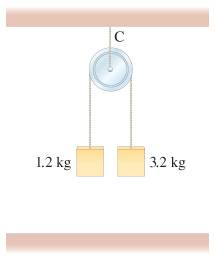Tension of string on pulley?

Homework Statement

Suppose the pulley is suspended by a cord C

Determine the tension in this cord after the masses are released and before one hits the ground. Ignore the mass of the pulley and cords.Mass 1 : 1.2kg
Mass 2 : 3.2kg
Pulley and String mass is negligible

Homework Equations

T-m1g = -m1a
T-m2g = m2a
Tension of pulley = T(C) -mg - 2T = ma(?)

The Attempt at a Solution

So I solved for the tensions in both ropes. Since they're equal I got T=17N
For acceleraton I got 4.4545455m/s. I'm just not too sure on the equation on the Tension of Cord C. Would it be the equation I mentioned above? When I use that I get an answer around 96. Is that right?

Doc Al
Mentor
No, it's much simpler than that. Since the pulley is massless, the sum of the forces on it must add to zero. (Note further that there is no mg term acting on the pulley.)

So it's just T(C) = 2T?

because I was thinking mg was referring to the total weight.

Doc Al
Mentor
So it's just T(C) = 2T?
Yep, that's all it is.
because I was thinking mg was referring to the total weight.
You are analyzing the pulley, so mg can only refer to the weight of the pulley, which is zero.

Thanks a bunch